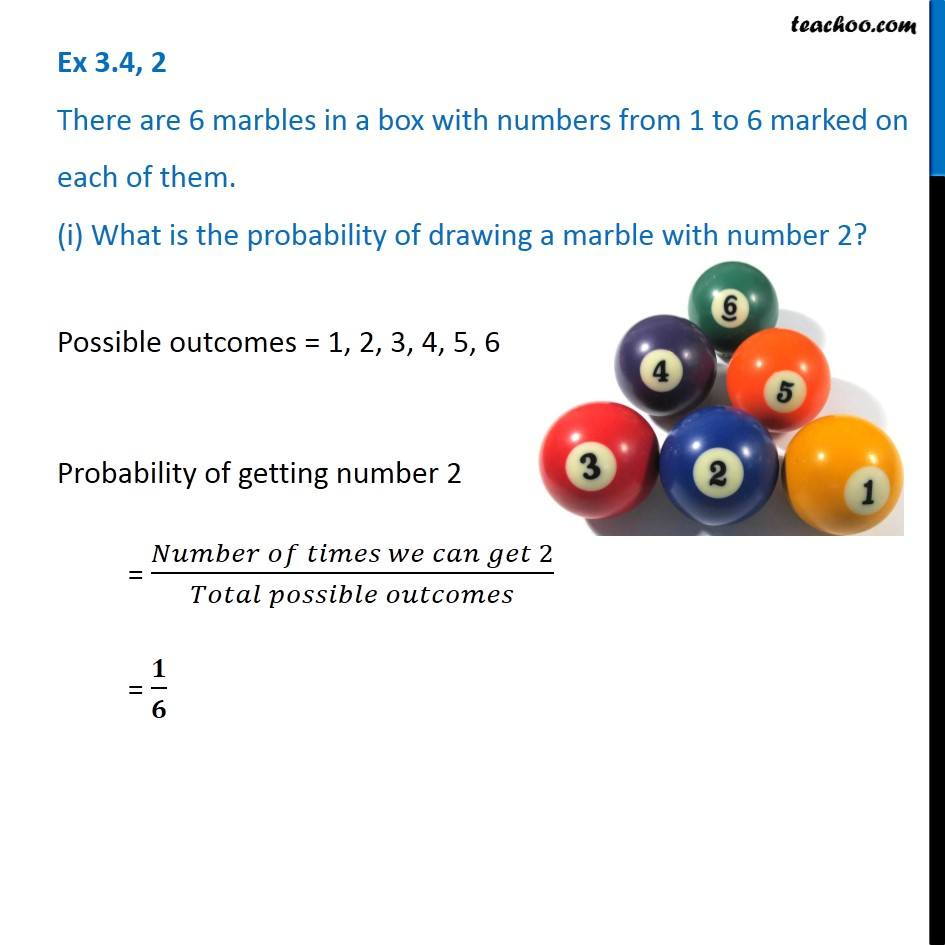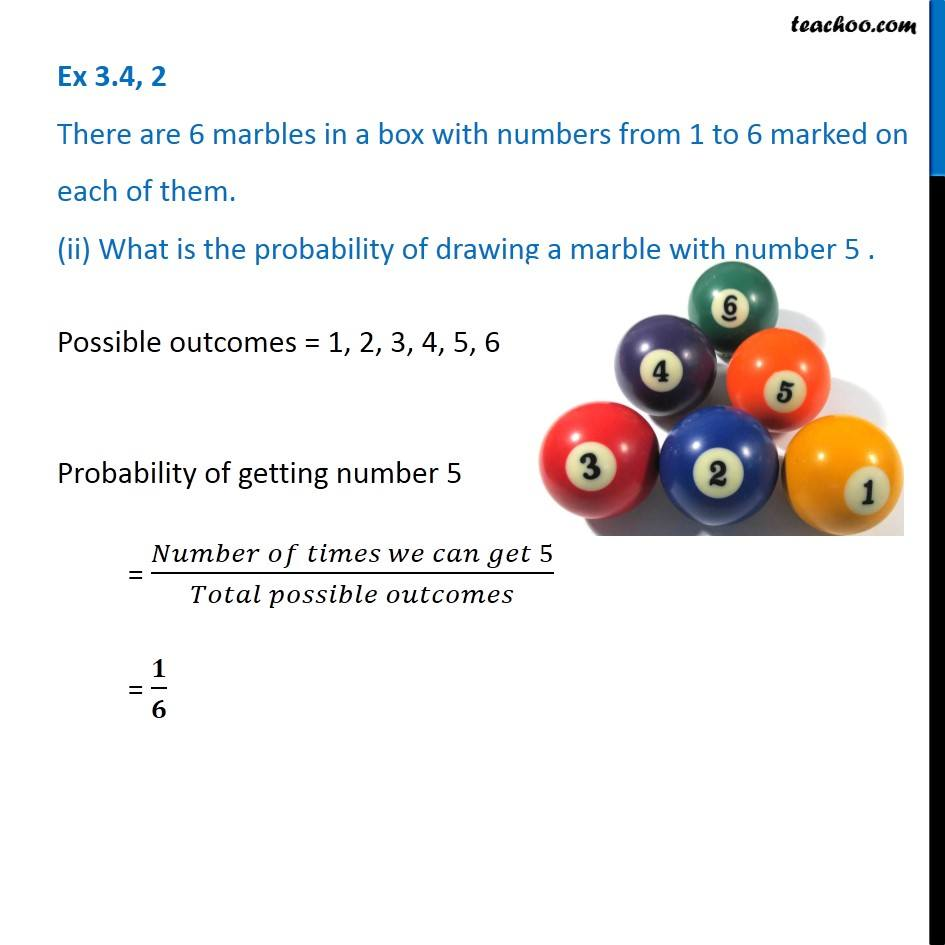Ex 3.4

Chapter 3 Class 7 Data Handling
Serial order wise### Transcript

Ex 3.4, 2 There are 6 marbles in a box with numbers from 1 to 6 marked on each of them. (i) What is the probability of drawing a marble with number 2? Possible outcomes = 1, 2, 3, 4, 5, 6 Probability of getting number 2 = (𝑁𝑢𝑚𝑏𝑒𝑟 𝑜𝑓 𝑡𝑖𝑚𝑒𝑠 𝑤𝑒 𝑐𝑎𝑛 𝑔𝑒𝑡 2)/(𝑇𝑜𝑡𝑎𝑙 𝑝𝑜𝑠𝑠𝑖𝑏𝑙𝑒 𝑜𝑢𝑡𝑐𝑜𝑚𝑒𝑠) = 𝟏/𝟔 Ex 3.4, 2 There are 6 marbles in a box with numbers from 1 to 6 marked on each of them. (ii) What is the probability of drawing a marble with number 5 . Possible outcomes = 1, 2, 3, 4, 5, 6 Probability of getting number 5 = (𝑁𝑢𝑚𝑏𝑒𝑟 𝑜𝑓 𝑡𝑖𝑚𝑒𝑠 𝑤𝑒 𝑐𝑎𝑛 𝑔𝑒𝑡 5)/(𝑇𝑜𝑡𝑎𝑙 𝑝𝑜𝑠𝑠𝑖𝑏𝑙𝑒 𝑜𝑢𝑡𝑐𝑜𝑚𝑒𝑠) = 𝟏/𝟔